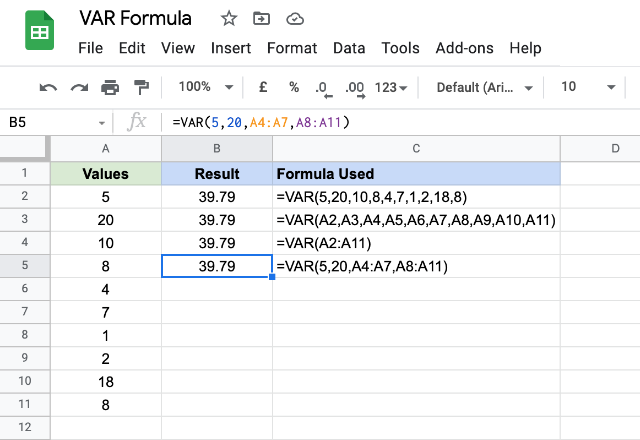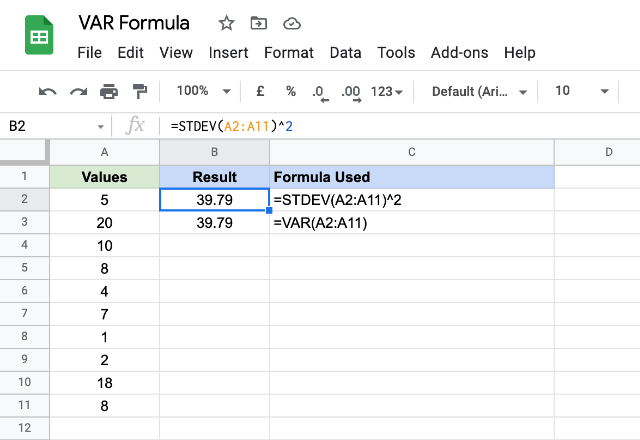# How to use the VAR formula in Google SheetsData is what the spreadsheets are built for – and helping us analyze it is its primary goal. There are some key metrics one needs to use while statistically analyzing the datasets. And variance is an important one of them. It measures how far a set of numbers are spread out from their average value. The VAR formula in Google Sheets is the corresponding readymade tool for us to be able to arrive at the variance of a dataset.

### Syntax

VAR(value1, [value2, …])

• value1 – is the very first value or the reference to the range of the sample.
• value2, … – these are sample’s additional values or references to the ranges.

### Usage: VAR formula in Google Sheets

Let us now consider a sample dataset and apply the formula practically so that we know what we are trying to accomplish. Please have a look at the following screenshot.If you are, by any chance, looking for some literature as to how we usually calculate variance, here’s the link to it.

The first example consumes direct numeric values and returns the calculated variance. The second demonstrates that the VAR formula is also capable of using references to cells that store numbers. In the third example, instead of sending multiple values or references, we have passed a single composite range. This composite cell range consists of all the values from A2 through A11. And, in the final example, we not only passed direct numeric values but also a couple range references. Therefore, regardless of the approach that we have taken with the formula, the output is same across all the examples.

#### Note

It is interesting to learn that the variance is nothing but the square of the standard deviation, another important metric in statistics. And we can we can obtain using the STDEV formula in Google Sheets. Please see the image that illustrates this concept.